# 5. Phi and Theta Extraction. Transform Method¶

Detailed description of all parameters and methods of BigARTM Python API classes can be found in Python Interface.

• Phi/Theta exraction

Let’s assume, that you have a data and a model, fitted on this data. You had tuned all necessary regularizers and used scores. But the set of quality measures of the library wasn’t enough for you, and you need to compute your own scores using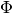and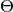matrices. In this case you are able to extract these matrices using next code:

```phi = model.get_phi()
theta = model.get_theta()
```

Note, that you need a `cache_theta` flag to be set True if you are planning to extractin the future without using `transform()`. You also can extract not whole matrices, but part of them, that corresponds different topics (using the same `topic_names` parameter of the methods, as in previous sections). Also you can extract only necessary modalities of thematrix, if you want.

Both methods return `pandas.DataFrame`.

• Transform new documents

Now we will go to the usage of fitted ready model for the classification of test data (to do it you need to have a fitted multimodal model with `@labels_class` modality, see 4. Multimodal Topic Models).

In the classification task you have the train data (the collection you used to train your model, where for each document the model knew it’s true class labels), and test one. For the test data true labels are known to you, but are unknown to the model. Model need to forecast these labels, using test documents, and your task is to compute the quality of the predictions by counting some metrics, AUC, for instance.

Computation of the AUC or any other quality measure is your task, we won’t do it. Instead, we will learn how to get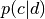vectors for each document, where each value is the probability of class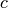in the given document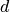.

Well, we have a model. We assume you put test documents into separate file in Vowpal Wabbit format, and created batches using it, which are covered by the variable `batch_vectorizer_test`. Also we assume you have saved your test batches into the separate directory (not into the one containing train batches).

Your test documents shouldn’t contain information about true labels (e.g. the Vowpal Wabbit file shouldn’t contain string ‘|@labels_class’), also text document shouldn’t contain tokens, that doesn’t appear in the train set. Such tokens will be ignored.

If all these conditions are met, we can use the `ARTM.transform()` method, that allows you to get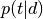(e.g.) ormatrix for all documents from your `BatchVectorizer` object.

Run this code to get:

```theta_test = model.transform(batch_vectorizer=batch_vectorizer_test)
```

And this one to achieve:

```p_cd_test = model.transform(batch_vectorizer=batch_vectorizer_test,
predict_class_id='@labels_class')
```

In this way you have got the predictions of the model in `pandas.DataFrame`. Now you can score the quality of the predictions of your model in all ways, you need.

Method allows you to extract dense od sparse matrix. Also you can use for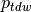matrix (see 8. Deal with Ptdw Matrix).

• Held-out perplexity calculation

To compute perplexity for held-out sample you should use the following code sample

```# create score if you haven't do so yet